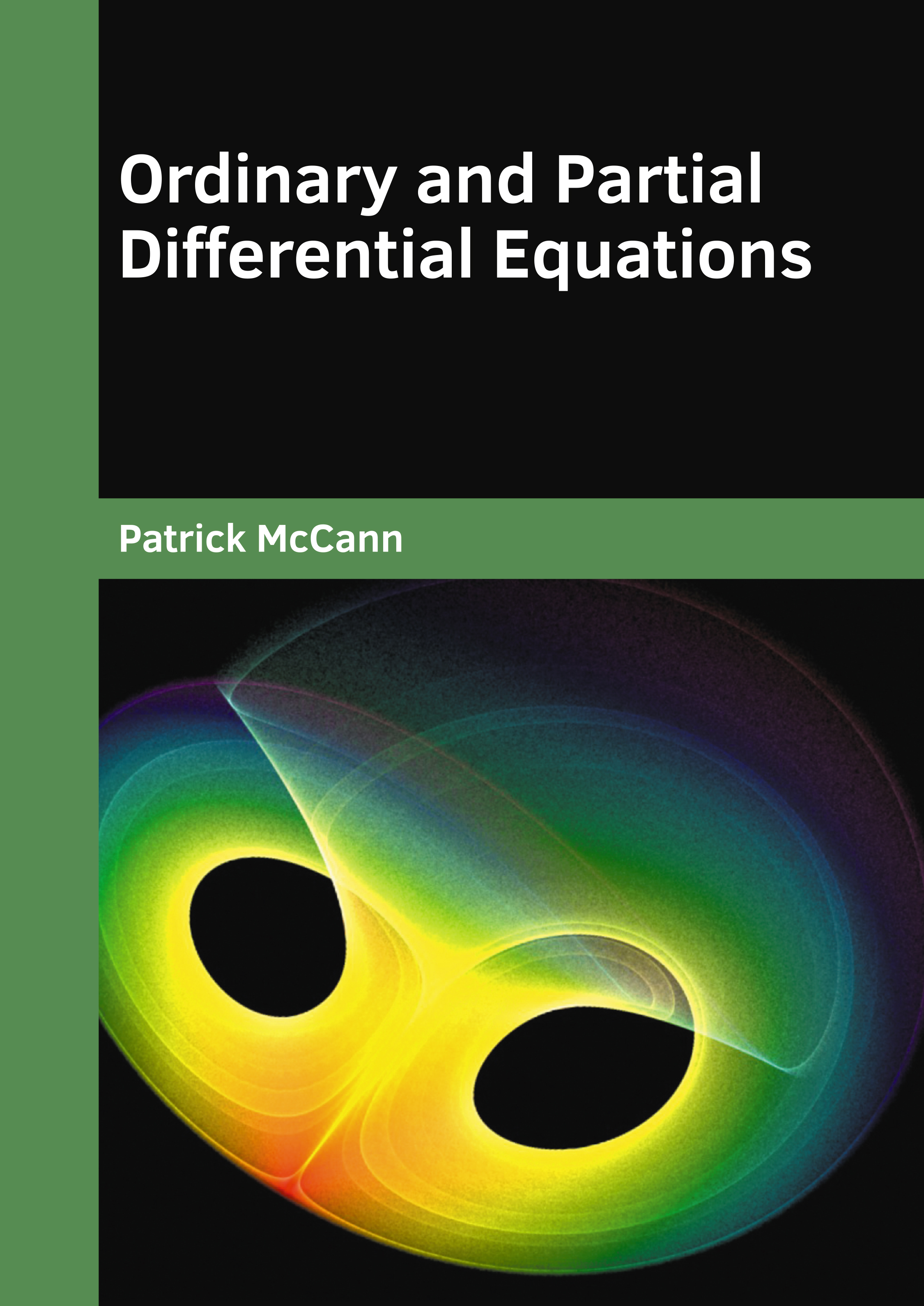BROWSE BY

#### Author

Results 1 - 1 of 1Ordinary and Partial Differential Equations Author : Patrick McCann Subject : Mathematics ISBN :9781682858240 The statement which expresses the equality of two expressions is known as an equation. A differential equation is a kind of mathematical equation that shows the connection between a function and its derivatives. Read More
Results 1 - 1 of 1